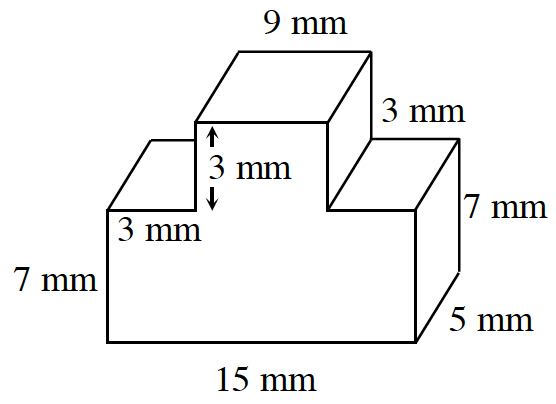### Home > MC2 > Chapter 11 > Lesson 11.2.2 > Problem11-45

11-45.

Find the volume of the prism at right. All angles are right angles.

Find the area of the base (which is the side facing you) and multiply by the height of $5$ mm.

The base can be divided into two rectangles. One that is $15$ mm by $7$ mm and one that is $9$ mm by $3$ mm.

Find the area of each smaller rectangle and add them together.

$\text{Volume of a prism = (area of base)(height)}$
Now multiply the area you found for the base by the height to get the total volume of the prism.

$660 \text{ mm}^3$